（日本語版はこちら

Depending on your job and hobbies, binary numbers might show up more or less often in your daily life. For many things like calculating subnet-masks, there are tools that do the work for you. For this reason, many people don't try to understand binary numbers in the first place.

This article is an attempt to simplify the binary system for people with little to no background in math or computer science, so the scary image doesn't hold you back from trying to understand it. Note that I try to avoid as much math as possible, or at least try to explain it in the most simple, but hopefully understandable way I can think of. If you are familiar with math, a lot of the following explanations might not be something new for you.

Now, for many calculations in IT related fields, it is enough to do calculations byte-wise, so I will focus on numbers no larger than 8 bit as examples.

### Just a little maths recap

We use terms as below:• Natural number
• Numbers that are used for counting with values 0 or more. E.g. 3, 15, 144 etc. are natural numbers, 1/3,  0.5, -4 are not.
• Power
• The result of multiplying the base (see graphic above) with itself repeatedly as often as the exponent (see graphic above) says, with the exponent being a natural number. E.g. 25 can also be written as 2×２×２×２×２.

### What are binary numbers?

The numbers we use in daily life are decimal numbers, meaning they consist of 10 different symbols, i.e. the digits 0-9. If you think about it, every number you can imagine is made out of a combination of these symbols. Now, numbers can also be expressed as binary numbers, meaning only using 2 different symbols, namely 0 and 1. Binary numbers are just a different way of writing the numbers you are used to in daily life using less symbols. This is useful for computers, as computers only understand the language of 0s and 1s, so numbers in binary form are just perfect for them.

For this article, we will only look at natural numbers, i.e. numbers having a value of 0 or more.

### Comparing binary and decimal numbers

Note: All binary numbers in this article are expressed in brackets with a small 2 at the end. E.g. (101)2 is the binary number 5, not the decimal number 101.Calculations with binary numbers are basically just the same as with decimal numbers. The only difference is how many symbols you can use per digit. So in order to understand binary numbers, let's first take a look at how decimal numbers work in detail.

Every decimal number can be split into its digits. For instance, let's look at the number 144. You can see it as the three digits 1, 4 and 4. Depending on the position within the actual number, each digit represents a multiple of a power of ten. What this means, is that 144 can also be expressed as 1 × 100 + 4 × 10 + 4 × 1, or shorter as 100 + 40 + 4 (see graphic below). Obviously 100 and 10 are powers of ten (100 = 102, 10 = 101), but for those of you who might not remember, 1 is a power of ten as well, as 1 = 100. It's good to remember, that the 0th power of any number is always 1. Even though it is obvious, it is also good to remember that a power of ten is always a 1 followed by zero or more 0s. You can get the exponent by counting the 0s, so e.g. 1000 = 103. Of course it works the other way around as well, if you have 10n you know it is a 1 followed by n 0s, e.g. 104 = 10000.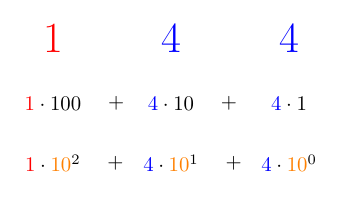Now, it's a good idea to convince yourself, that this works for any number you can think of. No matter what number you take, you will always end up with the digits as the factor to multiply the corresponding power of ten. In order to get the corresponding number of ten, again you just have to count the following digits respectively. Let's take 1607 as another example. This number can be split into 1 × 1000 + 6 × 100 + 0 × 10 + 7 × 1. 1 is followed by three digits, so the power of ten to multiply with is 1000 = 103; 6 is followed by two digits, so the power of ten to multiply with is 100 = 102, 7 is followed by zero digits, so the power of ten to multiply with is 1 = 100.

For binary numbers it's just the same idea!

Now, first we need to understand, that for binary numbers, a 1 followed by zero or more 0s is always a power of two. Again, given the number in binary form, you can get the exponent by counting trailing 0s. E.g., (1000)2 has three 0s, so we have 23 = 8.

Again, this works the same for every binary number. Let's take a look at the binary number (101)2. We can split it into its digits 1, 0 and 1, or just like above, into the form 1 × (100)2 + 0 × (10)2 + 1 × (1)2. So in this example, we have (100)2 = 22 = 4 and (1)2 = 20 = 1, so we get 4 + 1 = 5. If you have problems with calculating powers of two, just start with 1 and multiply it by 2 as many times as your exponent says, so in case of 22 you have 1 x 2 x 2 = 4.

What we have seen so far is that binary numbers really just work like decimal numbers, with the only difference being less symbols used per digit. Now let's see how that translates into a different kind of calculation.

If we add 1 to a decimal number, there are two different possible outcomes:

1. If the last digit was less than 9, we end up with the same number where the very last digit is increased by 1, e.g. 15 + 1 = 16.
2. If the last digit was 9, then we end up with a number where the last digit is now a 0 and the digit left to it is increased by 1, e.g. 139 + 1 = 140.

Note that for the increased digit the same two rules are being applied, depending on it being 9 or less than 9.

Now, this means, if we add 1 to a number that only consists of 9s, we will end up with a 1 followed by only 0s, which is of course a power of 10, e.g. 99 + 1 = 100. In other words, decimal numbers consisting only of 9s are a power of 10 - 1. This is because 9 is the maximum possible value for a digit in a decimal number. You will get the exponent by counting the 9s, e.g. 99 has two 9s, so 99 = 102 - 1.

So how about binary numbers? Note that the maximum possible value for each digit is 1. If you add 1 to a binary number, again there are two possible outcomes:

1. If the last digit was less than 1, i.e. 0, we end up with a number where the last digit is now 1 and the rest is unchanged, e.g. (110)2 + (1)2 = (111)2.
2. If the last digit was a 1, then we end up with a number where the last digit is now a 0 and the digit left to it is increased by 1, e.g. (101)2 + (1)2 = (110)2.

Let's look at the number (11)2 (= 3). If we add 1, we will get (100)2 (= 4), which is a power of 2. What this means is, that every binary number consisting of only 1s, is a power of 2 - 1. Even more so, by counting the 1s, you will again get the exponent. For instance, (111)2 has three 1s, so you get 23 - 1 = 8 - 1 = 7.

### Binary→Decimal

Now, if you see a binary number in the wild, what should you do in order to get the decimal expression of that very same number?

We will first do a method for plain calculation and get to tips and tricks for speeding calculations up later. So this is just a method that always works, no matter how small or big the number itself is. Basically we have just seen this method in the above paragraph, but for the sake of completeness here it is in a generalized form.

1. Whenever there is a 1 in your number, count the trailing digits (no matter if it's 0 or 1) in order to get the exponent. Let's call this number "n".
2. Calculate 2n
3. Add all the calculated numbers

Let's take a look at (10010000)2, where we have two 1s (see graphic below). Using the method described, we get 27 = 128 and 24 = 16. So, 128 + 16 = 144.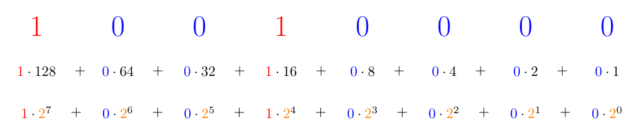### Decimal→Binary

Assuming we haven't memorized any powers of 2, probably the easiest way to express decimal numbers in binary is using Horner's method.

1. Divide your number by 2 (integer division) and mark down the remainder. (Either 0 or 1)
2. Take your result from 1. without the remainder and repeat the first step until it is 0
3. The marked down remainders from bottom to top (or right to left, depending on how you wrote it down) is your binary number!

So, let's say we want to express 144 in binary using Horner's algorithm.

1. 144 / 2 = 72, remainder: 0
2. 72 / 2 = 36, remainder: 0
3. 36 / 2 = 18, remainder: 0
4. 18 / 2 = 9, remainder: 0
5. 9 / 2 = 4, remainder: 1
6. 4 / 2 = 2, remainder: 0
7. 2 / 2 = 1, remainder: 0
8. 1 / 2 = 0, remainder: 1

As the result, we get 144 = (10010000)2.

### Basic tips and tricks

There are a lot of tricks that make calculations with binary numbers quicker.

• Memorizing 0-15 in binary
• This is very simple but helps a lot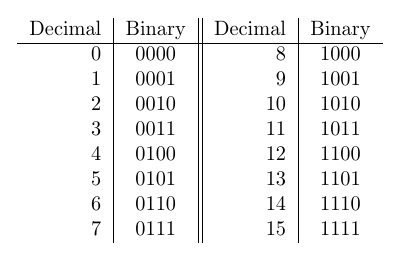• Memorizing powers of 2 up to 1024
• Again this is very simple, but comes in handy for all sorts of calculations. Even just recognizing a number as a power of 2 is very helpful. The game 2048 is very helpful for this!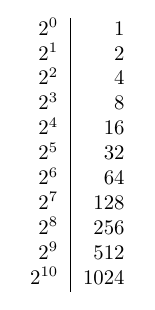• Appending a 0 at the end means multiplying by 2
• For instance, (11)2 -> (110)2 is 3 × 2 = 6
• Adding a 1 at the end means multiplying by 2 and adding 1
• For instance, (101)2 -> (1011)2 = 5 × 2 + 1 = 11
• The complement of a binary number with n digits is that number with all bits flipped, meaning all 1s turn into 0s and all 0s turn into 1s. These two numbers (the original number and its complement) added will be a binary number with n digits consisting only of 1s, thus having the value 2n - 1.
• 210 ≈ 1000 (1024 to be exact)
• 232 ≈ ４ billion
• You can practice counting in binary with your hand! Finger down means 0, finger up means 1. You can count from 0 to 31 using only one hand, and up to 1023 using both hands.

Tricks especially for bytes:

• Think in bytes
• Since byte-based calculations are very common, it helps a lot if you practice binary calculations with 8-digit numbers. Soon you will find patterns and become very quick at converting between binary and decimal.
• Memorize the powers of two at all positions within the byte (see graphic below)
• The right 4 digits are all 1-digit powers of 2, i.e. 1, 2, 4 and 8. This means higher-digit numbers start from the left of the middle. This trick is useful for spotting 16 and higher powers of 2 quickly.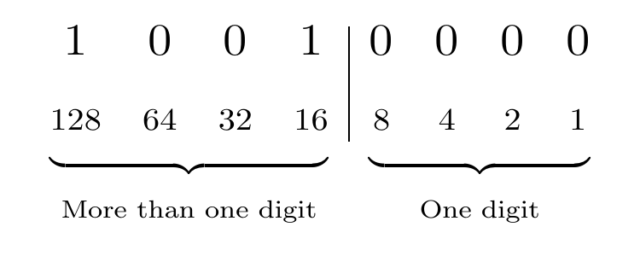### Characteristics at a glance

• All digits are 1s: power of 2 - 1
• Only one 1: power of 2
• Last digit is a 1: odd number
• In case of a byte: the value is never higher than 255

### Practical examples

Now let's look at some examples where binary numbers are actually used.

#### chmod

`chmod` sets the rights for a file or directory in Unix-like systems. The order of giving rights is Read - Write - Execute. Each of these rights is expressed using 0 (for deny) and 1 (for permit).

This means, that for instance full rights are expressed with `111`, which is 7 in binary. Another example is permission for reading and execution, but not for writing is expressed as `101`, which is 5 in binary. Now, these rights need to be set for the file-owner, the user-group and everyone else, in this order. Let's say we want to permit full rights to the user, but only read- and execute-permission to both the user-group and everyone else (meaning only the user can modify the file). We then use `chmod 755 some_file.txt` in order to do so.

If you've ever wondered what those chmod-numbers mean, now you know :)

IP-addresses consist of 4 octets divided by a dot, like this: `192.168.0.1`. Every octet is stored in a byte, so an IP-address has a total length of 4 bytes.

Since 1 byte is 8 bit (= 8 digit binary number including leading 0s), the values a single byte can take is from 0-255. Thus, every octet of an IP-address can only have a maximum value of 255. In order to reach the maximum value, all bits need to be set to 1.

Subnet masks are usually expressed like this: `192.168.0.1/24`.

The important part here is `/24`. This means, that 24 bits are used as the network-part of this IP-address. So this mask written out in dot-notation is 255.255.255.0, because the first 24 bits are set to 1.

The easiest subnet masks are `/8`, `/16``/24` and `/32`, because these numbers are multiples of 8. The corresponding subnet masks are just octets of value 255, followed by octets of value 0.

Now, for subnet masks of other lengths, you just need to look at the part that is the remainder after dividing by 8. So, if you have a subnet mask `/28`, you divide 28 by 8 and get 3 with a remainder of 4. This means, the first 3 octets are 255, and the first 4 bits of octet 4 are set to 1 (11110000). Now, depending on which way you prefer, you can either calculate 128 + 64 + 32 + 16 OR you can calculate the complement of that number, (00001111) which is 8 + 4 + 2 + 1 and subtract it from 255. In both cases you will get 240. Personally I prefer the second way, because it will always be a number only consisting of 1s (ignoring leading 0s), meaning you have a power of 2 minus 1, which is easily calculated (see tricks above).

ALL subnet masks are a string of 1s followed by zero or more 0s. This means, that the complementary number (for instance used in wildcard masks) is ALWAYS a power of 2 minus 1. This also means, that there is a very restricted number of possible subnet mask values. Considering only single octets instead of a whole IP-address, these values are:

• 255 (11111111)
• 254 (11111110) (basically not used, but possible)
• 252 (11111100)
• 248 (11111000)
• 240 (11110000)
• 224 (11100000)
• 192 (11000000)
• 128 (10000000)
• 0     (00000000)